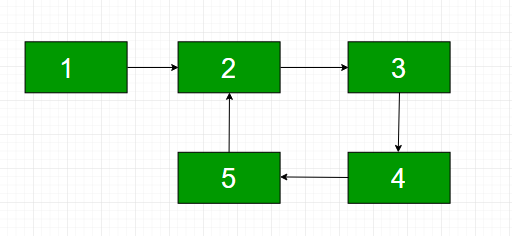# Find length of loop in a Linked List using Map

Write a program that checks whether a given Linked List contains loop and if a loop is present then returns the count of nodes in the loop. For example, a loop is present in the below-linked list and the length of the loop is 4. If the loop is not present, then the function should return 0.## Recommended: Please try your approach on {IDE} first, before moving on to the solution.

Approach: In this post, we will use the concept of Map to store addresses of nodes present in the linked list as a key and their position as the values.

Below is the step-by-step approach:

1. Traverse every node of the linked list and maintain the position starting with one. Increment the position after every node.
2. Check whether that node is present in the Map or not.
3. If the map does not contain the address of that node, insert it into the map along with its position.
4. If the map already contains the address of that node, return the difference between their positions.
5. If no such node has been found, return 0.

Below is the implementation of the above approach:

 `// C++ program to length of loop ` `// in a linked list using Map ` ` `  `#include ` `using` `namespace` `std; ` ` `  `// Linked List node ` `struct` `Node { ` `    ``int` `data; ` `    ``struct` `Node* next; ` ` `  `    ``Node(``int` `num) ` `    ``{ ` `        ``data = num; ` `        ``next = NULL; ` `    ``} ` `}; ` ` `  `// Function detects and counts loop ` `// nodes in the list. If loop is not there, ` `// then returns 0 ` `int` `countNodesinLoop(``struct` `Node* head) ` `{ ` `    ``struct` `Node* p = head; ` `    ``int` `pos = 0; ` ` `  `    ``// Maintain a map to store addresses ` `    ``// of node and their position ` `    ``unordered_map m; ` ` `  `    ``// Traverse through the linked list ` `    ``while` `(p != NULL) { ` ` `  `        ``// If the node is not present in the map ` `        ``if` `(m.find(p) == m.end()) { ` `            ``m[p] = pos; ` `            ``pos++; ` `        ``} ` ` `  `        ``// if the node is present ` `        ``else` `{ ` ` `  `            ``// Return difference between ` `            ``// position of the present node and ` `            ``// position where that node occured before ` `            ``return` `(pos - m[p]); ` `        ``} ` `        ``p = p->next; ` `    ``} ` ` `  `    ``// Return 0 to indicate ` `    ``// there is no loop ` `    ``return` `0; ` `} ` ` `  `// Driver code ` `int` `main() ` `{ ` `    ``// Create nodes of the linked list ` `    ``struct` `Node* head = ``new` `Node(1); ` `    ``head->next = ``new` `Node(2); ` `    ``head->next->next = ``new` `Node(3); ` `    ``head->next->next->next = ``new` `Node(4); ` `    ``head->next->next->next->next = ``new` `Node(5); ` ` `  `    ``// Create a loop for testing the function ` `    ``head->next->next->next->next->next = head->next; ` ` `  `    ``// Call the function for the above linked list ` `    ``cout << countNodesinLoop(head) << endl; ` ` `  `    ``return` `0; ` `} `

Output:

```4
```

Don’t stop now and take your learning to the next level. Learn all the important concepts of Data Structures and Algorithms with the help of the most trusted course: DSA Self Paced. Become industry ready at a student-friendly price.

My Personal Notes arrow_drop_upCheck out this Author's contributed articles.

If you like GeeksforGeeks and would like to contribute, you can also write an article using contribute.geeksforgeeks.org or mail your article to contribute@geeksforgeeks.org. See your article appearing on the GeeksforGeeks main page and help other Geeks.

Please Improve this article if you find anything incorrect by clicking on the "Improve Article" button below.

Article Tags :
Practice Tags :

3

Please write to us at contribute@geeksforgeeks.org to report any issue with the above content.